### IMO Shortlist 2018 problem G1

Kvaliteta:
Avg: 0,0
Težina:
Avg: 6,0

Let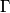$\Gamma$ be the circumcircle of acute triangle$ABC$. Points$D$ and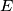$E$ are on segments$AB$ and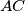$AC$ respectively such that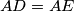$AD = AE$. The perpendicular bisectors of$BD$ and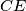$CE$ intersect minor arcs$AB$ and$AC$ of$\Gamma$ at points$F$ and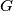$G$ respectively. Prove that lines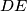$DE$ and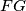$FG$ are either parallel or they are the same line.

Izvor: https://www.imo-official.org/problems/IMO2018SL.pdf